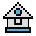Home

# CIRCLE Statement

#### Purpose:

To draw a circle, ellipse, and angles on the screen during use of the Graphics mode.

#### Syntax:

`CIRCLE(xcenter, ycenter), radius[,[color][,[start],[end][,aspect]]]`

xcenter and ycenter are the x- and y- coordinates of the center of the ellipse, and radius is the radius (measured along the major axis) of the ellipse. The quantities xcenter and ycenter can be expressions. The center attributes can use either absolute or relative coordinates.

color specifies the color of the ellipse. Its value depends on the current screen mode. See the COLOR and SCREEN statements for more information on using colors in different screen modes. In the high-resolution mode, 0 indicates black and 1 indicates white. The default for the high resolution mode is 1.

The start and end angle parameters are radian arguments between -2π and 2π which specify where the drawing of the ellipse is to begin and end. If start or end is negative, the ellipse is connected to the center point with a line, and the angles are treated as if they are positive (note that this is different from adding 2π).

aspect describes the ratio of the x radius to the y radius (x:y). The default aspect ratio depends on the screen mode, but gives a visual circle in either graphics mode, assuming a standard monitor screen aspect ratio of 4:3. If the aspect ratio is less than 1, then the radius is given in x-pixels. If it is greater than 1, the radius is given in y-pixels. In many cases, an aspect ratio of 1 gives better ellipses in the medium-resolution mode. This also causes the ellipse to be drawn faster. The start angle may be less than the end angle.

#### Example 1:

`10 SCREEN1: CIRCLE(100,100), 50`

Draws a circle of radius 50, centered at graphics points 100x and 100y.

#### Example 2:

```1 ' This will draw 17 ellipses
10 CLS
20 SCREEN 1
30 FOR R=160 TO 0 STEP-10
40 CIRCLE (160,100),R,,,,5/18
50 NEXT```

#### Example 3:

```10 'This will draw 5 spheres
20 GOTO 160
50 IF VERT GOTO 100
60 CIRCLE (X,Y),R,C,,,.07
70 FOR I = 1 TO 5
80 CIRCLE (X,Y),R,C,,,I*.2:NEXT I
90 IF VERT THEN RETURN
100 CIRCLE (X,Y),R,C,,,1.3
110 CIRCLE (X,Y),R,C,,,1.9
120 CIRCLE (X,Y),R,C,,,3.6
130 CIRCLE (X,Y),R,C,,,9.8
140 IF VERT GOTO 60
150 RETURN
160 CLS: SCREEN 1: COLOR 0,1: KEY OFF: VERT=0
170 X=160: Y=100: C=1: R=50: GOSUB 50
180 X=30: Y=30: C=2: R=30: GOSUB 50
190 X=30: Y=169: GOSUB 50
200 X=289: Y=30: GOSUB 50
210 X=289: Y=169: GOSUB 50
220 LINE (30,30)-(289,169),1
230 LINE (30,169)-(289,30),1
240 LINE (30,169)-(289,30),1,B
250 Z\$=INKEY\$: IF Z\$="" THEN 250```